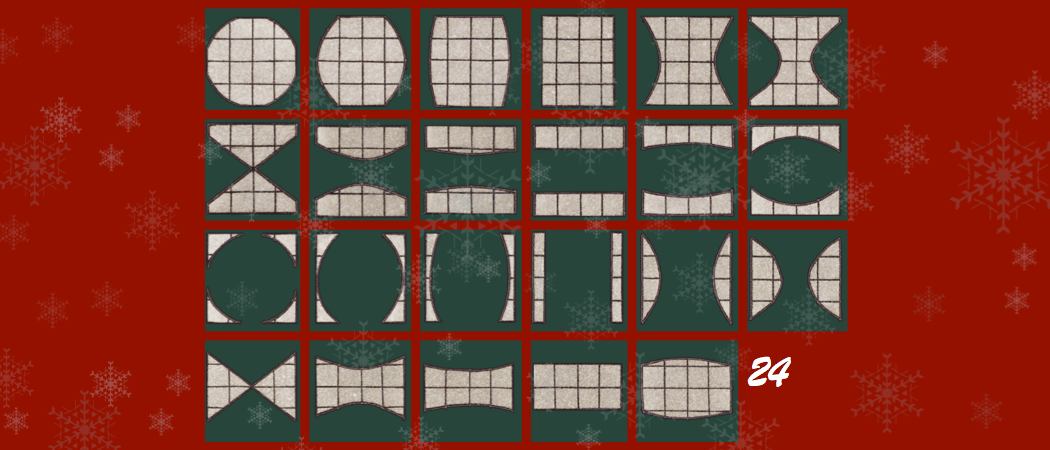Behind today’s door… a puzzle!This post was part of the Chalkdust 2016 Advent Calendar.

Welcome to the twenty third day of the 2016 Chalkdust Advent Calendar. Today, we have another puzzle for you to enjoy, plus the answer to the puzzles from 12 December.

Here’s today’s puzzle.

### Finding Santa

It’s Christmas Eve Eve and Santa is so nervous that he decides to hide in the top floor of a nearby hotel. Santa starts in a random room and every hour moves to one of the rooms next door to his current room. There are 17 rooms on the top floor of the hotel.

The hotel manager is a very busy lady and only has time to allow you to look into one room every hour. It’s 2pm on Christmas Eve Eve, so you have 34 hours to find Santa or Christmas is ruined. Can you find him in time?

Now for the solution to the puzzle from 12 December.

### Odd factors

Pick a number. Call it $n$. Write down all the numbers from $n+1$ to $2n$ (inclusive). Under each of these, write its largest odd factor. What is the sum of these odd factors?

Incredibly, the result will always be $n^2$.

To see why, imagine writing every number, $n+1\leq k\leq 2n$, in the form $$k=2^ab$$ where $b$ is an odd number and also the $k$’s largest odd factor. The next largest number whose largest odd factor is $b$ will be $2^{a+1}b=2k$. But this will be larger than $2n$, so outside the range. Therefore each number in the range has a different largest odd factor.

Each of the largest odd factors must be one of $1, 3, 5, …, 2n-1$, as they cannot be larger than $2n$. But there are $n$ odd numbers here and $n$ numbers in the range, so each number $1, 3, 5, …, 2n-1$ is the highest odd factor of one of the numbers (as the highest odd factors are all different).

Therefore, the sum of the odd factors is the sum of the first $n$ odd numbers, which is $n^2$.Matthew is a postdoctoral researcher at University College London. He hasn’t had time to play Klax since the noughties, but he’s pretty sure that Coke is it!